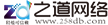# 监控杆的基础计算公式

###### 2017-11-10　来自: 沈阳鹏源灯具厂浏览次数:1062

⑵．自然条件：当地基本风压Wo=0.75KN/m2，地基土为淤泥质粘性土，地承载力特征值fak=60 KN/m2，地面粗糙度考虑城市郊区为B类，地下水位埋深大于2.5m，地基土的容重γm=18 KN/m3。
⑶．设计计算依据：   ①、《建筑结构荷载规范》 GB50009-2001   ②、《建筑地基基础设计规范》GB5007-2002   ③、《钢结构设计规范》  GB50017-2003   ④、《高耸结构设计规范》     GBJ135-90

μr —高耸结构重现期调整系数，对重要的高耸结构取1.2。   ⑴．灯盘：高度为30m，μz =1.42，μs =0.5，μr=1.2        βz=1+
式中ξ—脉动增大系数；      υ—脉动影响系数；     φz—振型系数；
βz=1+ =1+（ ）=2.04      WK=βz·μs·μz·ur·Wo       =2.04×0.5×1.42×1.2×0.75=1.30KN/m2   ⑵．灯杆：简化为均布荷载，高度取15m，     μz=1.4, μs=0.59, μr=1.2     βz=1+ =1+（ ）=2.16，     WK2=βz·μs·μz·ur·Wo      =2.16×0.59×1.14×1.2×0.75=1.31KN/m2 四、内力计算  ⑴．底部（δ=8mm）     弯矩设计值：M=M灯盘+M灯杆
M=γQ×WK1×0.2×3.8×30+γQ×WK2× ×30×15     =1.4×1.30×0.2×3.8×30+1.4×1.31× ×30×15     =426KN·m
剪力设计值：V=V灯盘+V灯杆
V =γQ×WK1×0.2×3.8+γQ×WK2× ×30     =1.4×1.30×0.2×3.8+1.4×1.31× ×30     =27KN
⑵．δ=8mm与δ=6mm，交接处     弯矩设计值：   M=γQ×WK1×0.2×3.8×10+γQ×WK2×（0.28+ ）×10×5     =1.4×1.30×0.2×3.8×10+1.4×1.31×（0.28+ ）×10×5     =51KN·m
剪力设计值：
V =γQ×WK1×0.2×3.8+γQ×WK2×（0.28+ ）×10     =1.4×1.30×0.2×3.8+1.4×1.31×（0.28+ ）×10     =9KN

最大剪应力τmax=2·V/A=2×27×103/[ ×（6502－6342）]=3.3N/mm2   max<f，Τmax

Gk=b×h×H×γ0=4×4×2.5×20=800KN
⑶．相应于荷载效应标准组合时，作用于基础底面的弯矩值：     Mk=M/γQ+VH/γQ        =426/1.4+27×2.5/1.4×2.5=353KN·m   ⑷．修正后的地基承载力特征值：      fa＝fak+ηb·γ(b-3)+ ηd·γm(d-0.5)      =60+0+1.0×18×(2.5－0.5)=96KN/m2
⑸．相应于荷载效应标准组合时，作用于基础底面边缘的最大最小压力值：     Pkmax= +
= =53+33=86KN/m2<1.2fa=115KN/m2能满足要求。     Pkmax= +
= =53－33=20KN/m2>0能满足要求。

⑵．高杆灯的安全等级，按高耸结构设计规范取一级。
⑶．基础设计所需的基本数据为：地基承载力特征值，地基土的物理性参数，上部荷载的标准组合值包括：弯矩、剪力、轴力。   ⑷．基础选型应根据建设场地土条件和结构的要求确定，一般为圆型混凝土独立基础或方形混凝土独立基础，底面积尺寸和配筋由计算确定。   ⑸．本文未复校立杆的稳定性，对立杆与基础连接也未作计算。各位读者自行设计计算

### 相关资讯更多>>

沈阳鹏源灯具厂（电话：13904050641）位于辽宁省沈阳市于洪区造化镇，本公司主要生产：沈阳监控杆沈阳交通信号杆沈阳交通指示牌杆沈阳路灯杆沈阳高杆灯，欢迎来电咨询。

更多关于：沈阳监控杆沈阳交通信号杆沈阳交通指示牌杆沈阳路灯杆沈阳高杆灯欢迎访问官方网站

公安备案链接：www.miitbeian.gov.cn

CopyRight © 版权所有: 沈阳鹏源灯具厂 技术支持:网站地图 XML 备案号:辽ICP备16003217号-1